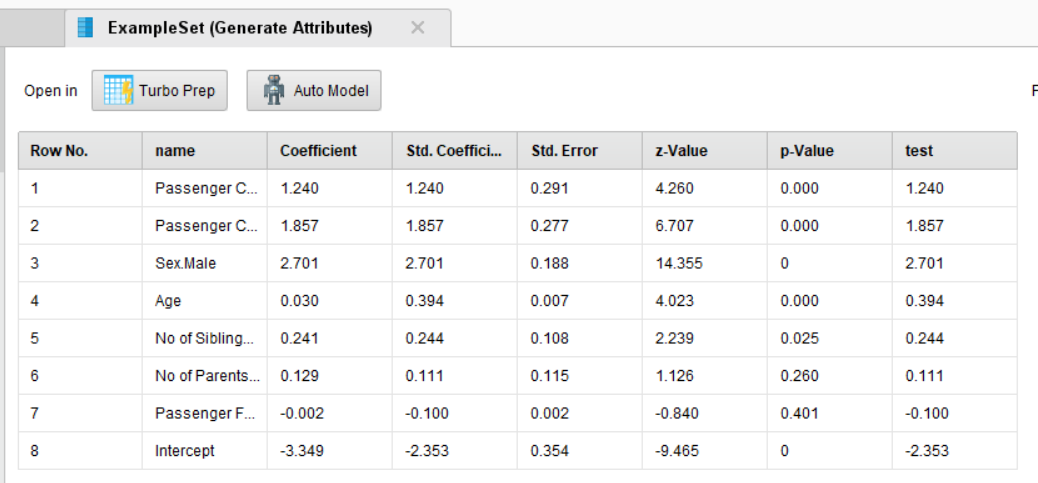# I need to convert model output to data !!

Member Posts: 8Contributor II
in Help
Hello everyone, I built a logistic regression model to get the coefficients and Intercepts to generate new attributes .. so how can I convert the result of this model to data so I can use it ?? Thanks

• Member Posts: 8Contributor II
professor @jmergler
, I just tried this, it creates a dataset from log Reg but with no data, so what is the issue?
• Moderator, Member Posts: 1,185Unicorn
edited May 2019
Hello @omarnj

Can you share your XML process? (View --> Show Panel --> XML) This extension will convert the coefficients into an example set as mentioned by @jmergler. Here is the tested process on titanic dataset.

```<?xml version="1.0" encoding="UTF-8"?><process version="9.2.001">
<context>
<input/>
<output/>
<macros/>
</context>
<operator activated="true" class="process" compatibility="9.2.001" expanded="true" name="Process">
<parameter key="logverbosity" value="init"/>
<parameter key="random_seed" value="2001"/>
<parameter key="send_mail" value="never"/>
<parameter key="process_duration_for_mail" value="30"/>
<parameter key="encoding" value="SYSTEM"/>
<process expanded="true">
<operator activated="true" class="retrieve" compatibility="9.2.001" expanded="true" height="68" name="Retrieve Titanic Training" width="90" x="179" y="34">
<parameter key="repository_entry" value="//Samples/data/Titanic Training"/>
</operator>
<operator activated="true" class="h2o:logistic_regression" compatibility="9.2.000" expanded="true" height="124" name="Logistic Regression" width="90" x="380" y="85">
<parameter key="solver" value="AUTO"/>
<parameter key="reproducible" value="false"/>
<parameter key="use_regularization" value="false"/>
<parameter key="lambda_search" value="false"/>
<parameter key="number_of_lambdas" value="0"/>
<parameter key="lambda_min_ratio" value="0.0"/>
<parameter key="early_stopping" value="true"/>
<parameter key="stopping_rounds" value="3"/>
<parameter key="stopping_tolerance" value="0.001"/>
<parameter key="standardize" value="true"/>
<parameter key="non-negative_coefficients" value="false"/>
<parameter key="compute_p-values" value="true"/>
<parameter key="remove_collinear_columns" value="true"/>
<parameter key="missing_values_handling" value="MeanImputation"/>
<parameter key="max_iterations" value="0"/>
<parameter key="max_runtime_seconds" value="0"/>
</operator>
<operator activated="true" class="converters:h2o_logreg_2_example_set" compatibility="0.5.000" expanded="true" height="82" name="Logistic Regression to ExampleSet" width="90" x="514" y="136"/>
<operator activated="true" class="generate_attributes" compatibility="9.2.001" expanded="true" height="82" name="Generate Attributes" width="90" x="648" y="136">
<list key="function_descriptions">
<parameter key="test" value="[Std. Coefficient]"/>
</list>
<parameter key="keep_all" value="true"/>
</operator>
<connect from_op="Retrieve Titanic Training" from_port="output" to_op="Logistic Regression" to_port="training set"/>
<connect from_op="Logistic Regression" from_port="model" to_op="Logistic Regression to ExampleSet" to_port="mod"/>
<connect from_op="Logistic Regression to ExampleSet" from_port="exa" to_op="Generate Attributes" to_port="example set input"/>
<connect from_op="Generate Attributes" from_port="example set output" to_port="result 1"/>
<portSpacing port="source_input 1" spacing="0"/>
<portSpacing port="sink_result 1" spacing="0"/>
<portSpacing port="sink_result 2" spacing="0"/>
</process>
</operator>
</process>
```

Here is the example set.Regards,
Varun
https://www.varunmandalapu.com/

# Be Safe. Follow precautions and Maintain Social Distancing

• Member Posts: 8Contributor II
thanks, @varunm1 , it works finally but I have another question now if you may help !! How can I use this data to make it matches each row in my data, so for example in Titanic Data , the coefficient of Sex.male is 2.701 .. so how can i match it with my data so all people who are male there is a column next with the coefficient of 2.701 .. hope my query is clear

thanks